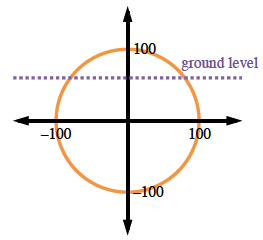### Home > CCA2 > Chapter Ch7 > Lesson 7.2.1 > Problem7-119

7-119.

The CPM Amusement Park has decided to imitate The Screamer but wants to make their ride even better. Their ride will consist of a circular track with a radius of 100 feet, and the center of the circle will be 50 feet under ground. Passengers will board at the highest point, so they will begin with a blood-curdling drop. Write a function that relates the angle traveled from the starting point to the height of the rider above or below the ground.

Begin by sketching a unit circle to model the situation.  When the passenger boards at $90^\circ$ on the unit circle (but $0^\circ$ traveled), they are $50$ feet above ground.

When they have traveled $90^\circ \left(\frac{\pi}{2}\text{ radians}\right)$ the passenger has moved to $50$ feet below ground, or the center point.

After traveling $180^\circ(\pi \text{ radians})$ they are $150$ feet below ground.

After moving $270^\circ \left(\frac{\pi}{2} \text{ radians}\right)$, they are back to $50$ feet above ground.

They return to $50$ feet above ground after turning a full circle, $360^\circ(2\pi \text{ radians})$.Make a table of values then sketch the graph.

 $x$(radians) $y$(feet) $0$ $50$ $\frac{\pi}{2}$ $-50$ ${\pi}$ $-150$ $\frac{3\pi}{2}$ $-50$ $2{\pi}$ $50$ $\frac{5\pi}{2}$

Determine the parameters of the equation.
As with any periodic function, either $y = \sin x$ or $y = \cos x$ could be the parent graph for the equation.
Since we have started at a high point, we will use cosine as the parent.
Determine the horizontal and vertical shifts and the amplitude.

The horizontal shift $\left(h\right)\ \text{is}\ 0$. The vertical shift (k) is $50$ feet down. The amplitude (a) is $100$.
Substitute the parameters into the general equation, $y = a · \sin\left(x − h\right) + k$.
Use a graphing calculator to see that your equation matches your graph.

$y = 100\text{sin} \left(x + \frac{\pi}{2}\right) - 50\text{ or }y = 100 \text{cos}(x) - 50$